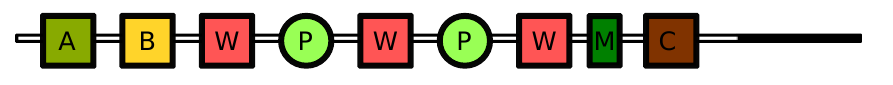### H - Kabobs

##### Languages: MS C# .NET 4.7.2053,GNU G++11 5.1.0 ...

Anna wants to open a marvelous restaurant, Candy Mountain'', serving only candy kabobs: sticks on which one puts various pieces of food, to be eaten from the tip to the base.

Like other kabob enthusiasts, when Anna eats a pattern of consecutive types of food in a kabob, she expects to eat another pattern later in the kabob. For instance, once she eats a piece of apple immediately followed by a piece of banana, she expects to have a leaf of mint immediately followed by chocolate in the remaining part of the kabob. She is happy if she finds this mint-chocolate pattern anywhere in the remaining kabob pieces.

Here is a kabob that Anna likes:
Apple-Banana-Watermelon-Plum-Watermelon-Plum-Watermelon-Mint-Chocolate

or, graphically:Anna has written down her perfect set of kabob patterns for the new restaurant, but she worries that these rules would give too many potential kabob types.  This set of patterns is written down as a ruleset, where each rule is of the form $b$ implies $e$ afterwards'', where $b$ and $e$ are non-empty sequences of characters, representing food pieces. A rule of the form $b\,\texttt{>}\,e$ with $b=b_1 \ldots b_k$ and $e=e_1 \ldots e_l$ means that, if the pattern $b$ is encountered in the kabob, then the kabob should also contain $e$ at some point after. Each $b_{i+1}$ must immediately follow $b_i$ to trigger the rule and similarly each $e_{j+1}$ must immediately follow $e_j$ to satisfy it, but $b_k$ and $e_1$ do not need to be consecutive. No food piece can appear more than once in a rule (i.e., there is no $i,j$ such that $e_j=b_i$ and no $i\neq j$ with $b_i=b_j$ or $e_i=e_j$) but a food piece can appear in several rules.

Note that if there are several occurrences of the word $b$ in a kabob, they all need to be followed by an $e$. This can be a single $e$, as long as this $e$ appears after all the $b$.

In a ruleset, rules are separated by | and are of the form $u\,\texttt{>}\,v$ meaning that each pattern $u$ implies a pattern $v$ afterwards. $u$ and $v$ are words composed of alphanumerical characters and no character can appear twice in a rule. For instance, the ruleset AB>X|R>A|T>B describes three rules:

• for each $\texttt{AB}$ there must be an $\texttt{X}$ afterwards;
• for each $\texttt{R}$ there must be an $\texttt{A}$ afterwards; and
• for each $\texttt{T}$ there must be a $\texttt{B}$ afterwards.

Using this ruleset, the kabobs $\texttt{SBSB}$, $\texttt{REA}$, $\texttt{ABX}$, $\texttt{BA}$, $\texttt{ABXBA}$, $\texttt{RRA}$, $\texttt{TBTB}$, and $\texttt{RTABX}$ are valid; but $\texttt{RAT}$, $\texttt{TAB}$, and $\texttt{ABXAB}$ are not.

Anna asks you how many kabobs of a given size are compatible with her ruleset.

#### Input

• The first line contains an integer $K$, the size of all kabobs, followed with a space, and a non-empty string $S$ of alphanumerical characters ($\texttt{A}$' to $\texttt{Z}$', $\texttt{a}$' to $\texttt{z}$', and $\texttt{0}$' to $\texttt{9}$'), representing the elements that can be used in a kabob (no characters appear twice in $S$).
• The second line contains a non-empty string $R$, which contains no spaces and represents a ruleset as described above. The patterns in $R$ are composed of characters from $S$ only.

Limits
The input is such that $1 \leq K \leq 500$ and $3\leq |R| \leq 60$.

#### Output

One integer: the number of kabobs of length $K$ satisfying all the rules in $R$, modulo $10\,000\,000$.

#### Sample test(s)

Input
4 ABC A>B|B>C|CB>A
Output
9